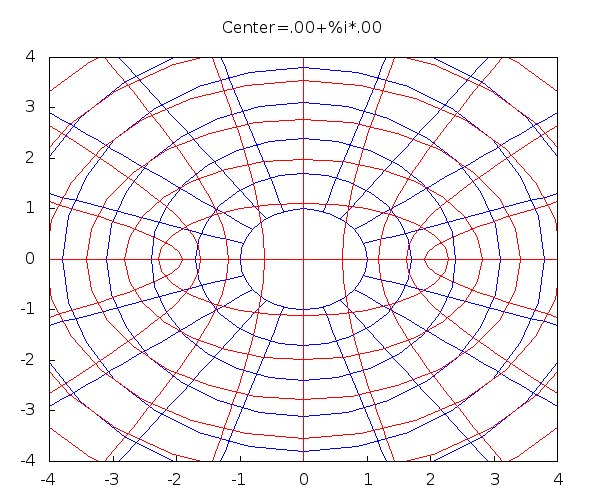From the catalogue:
Complex functions and integration, line and surface integrals, Fourier series, partial differential equations.

All visualization below are done using Maxima computer algebra system (CAS).

## Cart simulation

This is a simulation of a differential equation for the displacement $y=y(t)$ of a cart ensemble, consisting of a cart of mass $m$, a spring with spring constant $k$ and a damper with damping constant $b$. $m\,y'' + b\,y'+k\,y = f(t)$ where $f(t)=f_0\,\sin(2\pi\omega\,t)$ i.e. the cart is subjected to an external periodic force.## Joukovski map

This is the conformal map: $J(z)=z+\frac{1}{z}$ Shown is the mapping the circle $|z-a|=|1-a|$ (blue) to an airfoil (red). The number $a$ (the center) varies over an interval in the complex domain.## D'Alembert's Solution of the Wave Equation with Maxima

### String plucked in the middle### String plucked at 20% of the string length